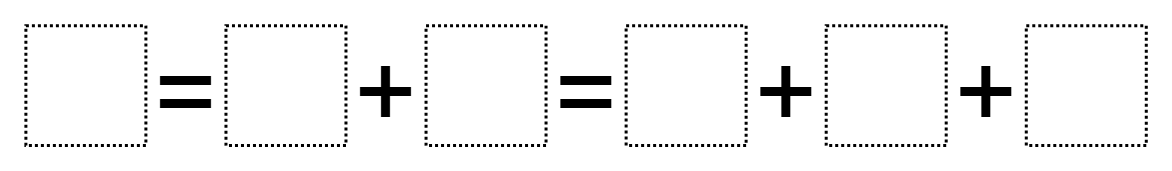Home > Grade 1 > Make it Equal

# Make it Equal

Directions: Place digits 1 through 9 in the boxes to create a true statement. Each number can only be used once.### Hint

Are there numbers that are helpful to use first or save for last?
What would a drawing/model look like to match this equation?

### Answer

Number of Unique Solutions: 48 (allowing for permutations)
1: 8 = 2+6 = 1+3+4
2: 8 = 2+6 = 1+4+3
3: 8 = 2+6 = 3+1+4
4: 8 = 2+6 = 3+4+1
5: 8 = 2+6 = 4+1+3
6: 8 = 2+6 = 4+3+1
7: 8 = 6+2 = 1+3+4
8: 8 = 6+2 = 1+4+3
9: 8 = 6+2 = 3+1+4
10: 8 = 6+2 = 3+4+1
11: 8 = 6+2 = 4+1+3
12: 8 = 6+2 = 4+3+1
13: 9 = 1+8 = 2+3+4
14: 9 = 1+8 = 2+4+3
15: 9 = 1+8 = 3+2+4
16: 9 = 1+8 = 3+4+2
17: 9 = 1+8 = 4+2+3
18: 9 = 1+8 = 4+3+2
19: 9 = 2+7 = 1+3+5
20: 9 = 2+7 = 1+5+3
21: 9 = 2+7 = 3+1+5
22: 9 = 2+7 = 3+5+1
23: 9 = 2+7 = 5+1+3
24: 9 = 2+7 = 5+3+1
25: 9 = 4+5 = 1+2+6
26: 9 = 4+5 = 1+6+2
27: 9 = 4+5 = 2+1+6
28: 9 = 4+5 = 2+6+1
29: 9 = 4+5 = 6+1+2
30: 9 = 4+5 = 6+2+1
31: 9 = 5+4 = 1+2+6
32: 9 = 5+4 = 1+6+2
33: 9 = 5+4 = 2+1+6
34: 9 = 5+4 = 2+6+1
35: 9 = 5+4 = 6+1+2
36: 9 = 5+4 = 6+2+1
37: 9 = 7+2 = 1+3+5
38: 9 = 7+2 = 1+5+3
39: 9 = 7+2 = 3+1+5
40: 9 = 7+2 = 3+5+1
41: 9 = 7+2 = 5+1+3
42: 9 = 7+2 = 5+3+1
43: 9 = 8+1 = 2+3+4
44: 9 = 8+1 = 2+4+3
45: 9 = 8+1 = 3+2+4
46: 9 = 8+1 = 3+4+2
47: 9 = 8+1 = 4+2+3
48: 9 = 8+1 = 4+3+2

Source: Molly Rawding

## Adding with Parenthesis

Directions: Using the digits 1 to 9, at most one time each, make a true …

### 3 comments

1.Okay, I am going to be THAT person right now. But I just noticed that the second example answer uses the digit 1 twice. Just attending to precision, over here. 🙂
This is such an awesome activity. My students blow me away with their engagement and perseverance in looking for multiple ways to solve! Thanks, as always, for sharing these fantastic resources.

2.The first answer is wrong . It should be 9=7+2=5+3+1

3.Yes, definitely mistakes! Thank for catching that!
8 = 6 + 2 = 4 + 3 + 1
9 = 8 + 1 = 4 + 3 + 2